### Chemical Shift

If all 1H or 13C nuclei absorbed energy at exactly the same resonance frequency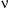, NMR spectroscopy would not be very useful for determination of organic and biochemical structures. However, different 1H or 13C nuclei absorb at slightly different frequencies. This difference results from differences in the small internal magnetic field Be for each 1H or 13C nucleus. Again, the Be field results from the circulation of electrons of the molecule. Different 1H or 13C nuclei have different electron density surrounding them and consequently, are shielded from the applied field Bo by Be fields of different magnitude. Remember, differences in (Bo-Be) translate into differences in the frequencyneeded to cause the nuclear spin transitions as shown by Equation 2.

The frequency of absorption for a nucleus of interest relative to the frequency of absorption of a molecular standard is called the chemical shift of the nucleus. The molecular standard for both 1H and 13C NMR spectroscopy is tetramethylsilane (TMS). Because of molecular symmetry all 12 protons of TMS absorb at the same frequency and all 4 carbons absorb at the same frequency.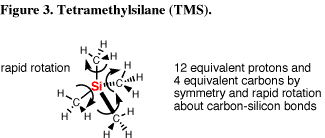In the NMR spectrum of an organic compound, peaks appear at the positions of absorption, also called the positions of resonance, also called the positions of resonance or precession frequencies, for different nuclei in the molecule. The exact chemical shift of a particular nucleus in a molecule gives us information about how the atom with that nucleus is bonded in the molecule. The x-axis of the spectrum is called the delta scale () with units of ppm and the y-axis is an intensity scale. The area under the peak on the y-axis is proportional to the number of 1H nuclei in the molecule with the same chemical shift. The intensity of a 13C resonance is, however, not proportional to the number of carbons and this will be discussed later.

On thescale, the position of resonance is given by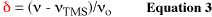where

• (-TMS) is the frequency difference between the resonance of the signal of interest and the resonance of TMS. Note that (-TMS) will always be in units of Hz (from 1 Hz to a few thousand Hz).
•is the center frequency of the B1 field in megahertz (MHz).
• If Bo = 7.05 T,= 300 MHz for 1H nuclei or 75 MHz for 13C nuclei.
• If Bo = 11.75 T,= 500 MHz for 1H nuclei or 125 MHz for 13C nuclei.

In Equation 3,is a ratio of Hz/MHz, the result is a ratio without units (1/1x106) or one over one million. A better term for "one over one millionth" is parts-per-million or ppm.

As a result of Equation 3 the chemical shift of the 1H nuclei in the 1H NMR spectrum or 13C nuclei in the 13C NMR spectrum of TMS appear at= 0 ppm. Typically,increases from 0 on the right hand side of the spectrum to 10 ppm on the left hand side of an 1H NMR spectrum or from 0 on the right hand side to 200 ppm on the left hand side of a 13C NMR spectrum. The reason frequencies of absorption are recorded on the-scale relative to those of a standard molecule is so the position of absorption will be independent of the spectrometer used to record the spectrum, in particular independent of the strength of the Bo field of the spectrometer. For example, the chemical shift of the protons of acetone will be the same (2.16 ppm), independent of whether the spectrum is obtained at 300 MHz or 500 MHz.

In principle, resonance can be achieved by holding Bo constant and scanning the frequency of the radio frequency excitation field B1. Alternatively, the frequency can be held constant and the strength of Bo can be scanned. In others words, equation 2 can be satisfied by varying the frequency of B1 or the strength of Bo for each different 1H or 13C nucleus in a molecule. In practice, modern NMR spectrometers operate at constant Bo.

The direction of increasing frequency at fixed field and increasing field at fixed frequency are shown on the following NMR chart paper (Figure 4). The right hand side is described as the high field region and the left hand side as the low field region.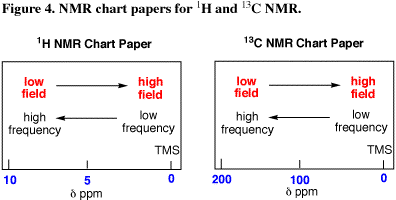The 1H NMR spectrum of methyl acetate shows two peaks: one at= 2.1 ppm for the three equivalent methyl protons and one at= 3.7 ppm for the three equivalent methoxy protons. The methoxy protons are said to resonate downfield from the methyl protons. Each of the three methyl protons are equivalent to each other and each of the three methoxy protons are equivalent to each because of rapid rotation about the carbon-carbon and carbon-oxygen single bonds. 1H or 13C nuclei are said to be chemical shift equivalent if they have exactly the same chemical shift; in other words they experience the same Be field. This most commonly results from molecular symmetry coupled with rapid rotations about single bonds, but can also occur by coincidence.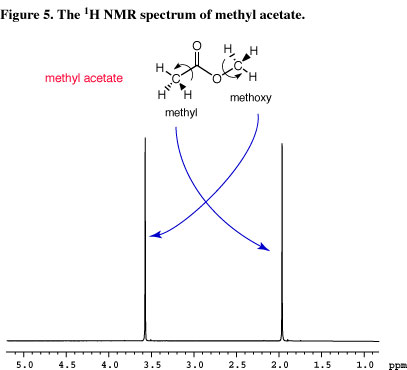The following is a summary of magnetic fields and frequencies. For your convenience, this and other reference information is included in the Reference section of this tutorial.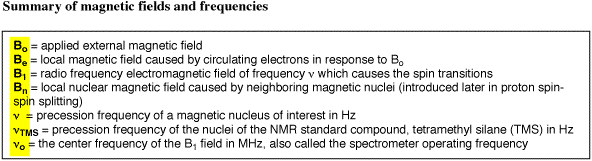next section: Chemical Shift - ProtonCopyright information: Original content © University of Colorado, Boulder, Chemistry and Biochemistry Department, 2011. The information on these pages is available for academic use without restriction.